Chaos and Complexity.
In the previous blog we discussed the growth of interest in deterministic chaos in low-dimensional dynamical systems, and the way in which it impinged on turbulence theory. Altogether, it seemed like a paradigm shift; in that we learned that only quantum effects were truly random, and that all classical effects were deterministic. If one knew the initial conditions of a classical dynamical system then one could, in principle, predict its entire evolution in time. If! Anyway, in those days we began to refer to the turbulent velocity field as being chaotic rather than random; but I suspect that the majority are now back to random.

However, such ideas also arose in the late 19th Century, as part of the invention of Statistical Mechanics, with Boltzmann’s assumption of molecular chaos. This was to the effect that molecular motion was uncorrelated immediately before and after a two-body collision. It was made in the context of a gas modelled as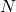particles in a box (whereis of the order of Avogadro’s number), and the motion of the particles is governed by Hamilton’s equations. The system can be specified by the-particle distribution (or density) which is the solution of Liouville’s equation. Although an exact formulation, this theory is contrary to experience because the entropy is found to be constant in time, which contradicts the second law of thermodynamics. This result is a well-known paradox and it was resolved by Boltzmann in his famous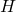-theorem.

Boltzmann wrote the entropy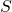in terms of a measureof the information about the system, thus: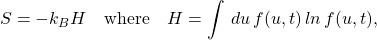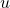is the molecular speed and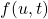is the single-particle distribution of molecular speeds. In obtaining the equation for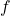, Boltzmann had to overcome a closure problem (much as in turbulence!) and his principle of molecular chaos justified the factorization of the two-particle function into the product of two single-particle functions. So Boltzmann’s-theorem is thatdecreases with time, meaning that the entropy increases.

Although this is not so well known, the paradox was also resolved by Gibbs, albeit in a more fundamental way. He showed that if a small amount of the information was lost from, then it was no longer invariant and would increase with time. In his case, this was achieved by coarse-graining the exact Liouville distribution function so that it was no longer exact, but it could equally well be achieved in practice by a slight deficiency in our specification of the initial conditions (position and momentum) of each of theparticles. In fact, to put it bluntly, it would be difficult (or perhaps impossible) to specify the initial state of a system of order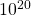degrees of freedom with sufficient accuracy to avoid the system entropy increasing with time.

The point to be taken from all this is that Hamilton’s equations, although themselves reversible in time, can nevertheless describe a real system which has properties that are not reversible in time. The answer lies in the complexity of the system.
This applies just as much to the quantum form of Hamilton’s equations. Recently there has been an international discussion (by virtual means) of the Einstein-Podolsky-Rosen paradox, which asserts that quantum mechanics is not a complete theory. I read some of the contributions to this, but was not impressed. In particular the suggestion was put forward that the time-reversal symmetry of the basic quantum equations of motion, ruled out their ability to describe a real world which undergoes irreversible changes; something that is generally referred to as ‘time’s arrow’. But of course the same applies to the classical form of the equations, and one must bear in mind that one has to take not only large-limits but also continuum limits to describe the real world.

There are lessons here, at least in principle, for turbulence theorists too, and I have given specific instances such as the irrelevance of intermittency or the incorrectness of the Onsager conjecture (when judged by the physics). No doubt I will give more in the months to come. Background material for this blog can be found in the lecture notes , which can be downloaded free of charge.

 W. David McComb. Study Notes for Statistical Physics: A concise, unified overview of the subject. Bookboon, 2014. (Free download of pdf from Bookboon.com)